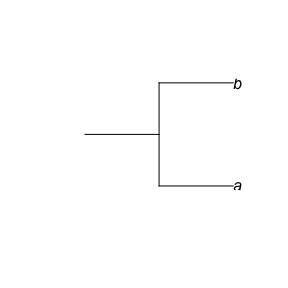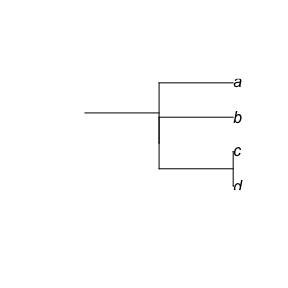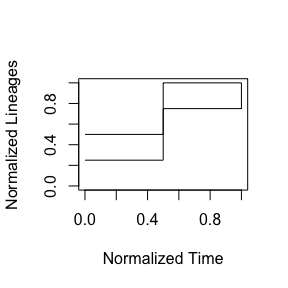# Close to polytomies, easier to eyeball

In ASCII art, I show two simple LTT plots:

# normalized number of lineages
#
# 1.0 +   X---X                                            #nolint
#     |   |
# 0.5 X---+                                                #nolint
#     |
#     +---+---+
#        0.5 1.0   normalized time
#
#
#
# normalized number of lineages
#
##  1.0  +       X                                         #nolint
##  0.75 |   X---+
##  0.5  |   |
##  0.25 X---+
##       +---+---+
##         0.5 1.0   normalized time

The nLTT statistic (simply the difference between the two) will be around 0.25.

This vignette shows how to measure this, from:

• two phylogenies
• two LTT plots
• two nLTT plots

## Two pylogenies

The two phylogenies

#
#          +---+ a
#      +---+
#          +---+ b
#
#      +---+---+
#     -2  -1   0 time (million years)
#
#
#
#           +---+ a
#       +---+---+ b
#           +---+ c
#               + d
#
#       +---+---+
#      -2  -1   0 time (million years)
#

First create those phylogenies:

phylogeny_1 <- ape::read.tree(text = "(a:1,b:1):1;")
text = "(((d:0.000000001,c:0.000000001):1,b:1):0.000000001,a:1.000000001):1;")
ape::plot.phylo(phylogeny_1, root.edge = TRUE)ape::plot.phylo(phylogeny_2, root.edge = TRUE)Plot their nLTTs (as gray) (and the average as black):

nLTT::nltt_plot(phylogeny_1, ylim = c(0, 1)); nLTT::nltt_lines(phylogeny_2)From this plot it can be estimated that the area between the two gray lines is 0.25. This means that the nLTT statistic is 0.25.

nltt_stat <- nLTT::nltt_diff(phylogeny_1, phylogeny_2)
nltt_stat_exact <- nLTT::nltt_diff_exact(phylogeny_1, phylogeny_2)
testthat::expect_equal(nltt_stat, 0.25, tolerance = 0.0001)
testthat::expect_equal(nltt_stat_exact, 0.25, tolerance = 0.0001)

## Two LTT plots

These are the original LTTs:

# normalized number of lineages
#
#  1.0 +   X---X
#      |   |
#  0.5 X---+
#      |
#      +---+---+
#         0.5 1.0   normalized time
#
#
#
# normalized number of lineages
#
#  1.0  +       X
#  0.75 |   X---+
#  0.5  |   |
#  0.25 X---+
#       +---+---+
#         0.5 1.0   normalized time

These are the LTTs:

# number of lineages
#
#    2 +   X---X
#      |   |
#    1 X---+
#      |
#    0 +---+---+
#      2   1   0   time in the past
#
#
# number of lineages
#
#    4  +       X
#    3  |   X---+
#    2  |   |
#    1  X---+
#    0  +---+---+
#       2   1   0   time in the past

I choose to use ‘time in the past’ as a time unit, as this is what ape does as well.

Here we extract the branching times and number of lineages from the phylogenies:

b_times <- nLTT::get_branching_times(phylogeny_1)
lineages <- nLTT::get_n_lineages(phylogeny_1)
b_times2 <- nLTT::get_branching_times(phylogeny_2)
lineages2 <- nLTT::get_n_lineages(phylogeny_2)
df <- data.frame(b_times = b_times, lineages = lineages)
knitr::kable(df)
b_times lineages
2 1
1 2
df2 <- data.frame(b_times2 = b_times2, lineages2 = lineages2)
knitr::kable(df2)
b_times2 lineages2
2 1
1 2
1 3
0 4

Here we test again if the nLTT statistic is 0.25:

nltt_stat_exact <- nLTT::nltt_diff_exact_brts(
b_times = b_times,
lineages = lineages,
b_times2 = b_times2,
lineages2 = lineages2,
distance_method = "abs",
time_unit = "ago")

testthat::expect_equal(nltt_stat_exact, 0.25, tolerance = 0.0001)

## Two nLTT plots

These are the original nLTTs:

# normalized number of lineages
#
#  1.0 +   X---X
#      |   |
#  0.5 X---+
#      |
#      +---+---+
#     0.0 0.5 1.0   normalized time
#
#
#
# normalized number of lineages
#
#  1.00 +       X
#  0.75 |   X---+
#  0.50 |   |
#  0.25 X---+
#  0.00 +---+---+
#      0.0 0.5 1.0   normalized time

I choose to use ‘time in the past’ as a time unit, as this is what ape does as well.

Here we extract the branching times and number of lineages from the phylogenies:

b_times_n <- nLTT::get_norm_brts(phylogeny_1)
lineages_n <- nLTT::get_norm_n(phylogeny_1)
b_times2_n <- nLTT::get_norm_brts(phylogeny_2)
lineages2_n <- nLTT::get_norm_n(phylogeny_2)
df <- data.frame(b_times_n = b_times_n, lineages_n = lineages_n)
knitr::kable(df)
b_times_n lineages_n
0 0
1 1
1 1
df2 <- data.frame(b_times2_n = b_times2_n, lineages2_n = lineages2_n)
knitr::kable(df2)
b_times2_n lineages2_n
0.0 0.0000000
0.5 0.3333333
0.5 0.6666667
1.0 1.0000000
1.0 1.0000000